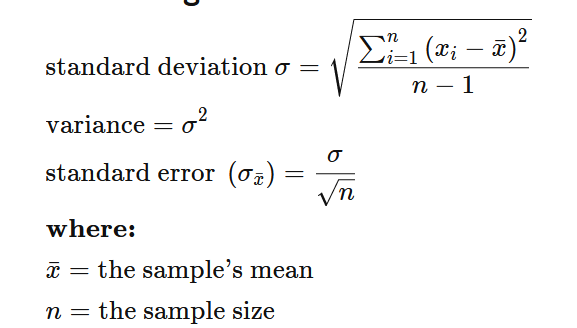# How the error of the standard distribution is calculated in root?

Hi,

I wonder how the errors of the standard deviation of a histogram are calculated in root?

Say for example, in the following histogram (TH1D*h1 = new TH1D(“h1”,“Au_158.62_MeV”,101.,39.5,140.5) attached :

Here the estimation of the mean, error in the mean and the standard deviation are straightforward using the following equation :But I don’t understand how the error in the standard deviation is calculated by root. Any help appreciated. Thank you.

Hi! Thanks for the question.

the uncertainty for the standard deviation is calculated as \sqrt{\frac{\sigma^2}{2n}}, which is the right estimator if the underlying distribution is Gaussian. For a general distribution this is not exact, because the right formula requires the 4th momentum of the distribution, which is not available to the histogram because of the binning.

I got this information from the source code:

This should probably also be written in the docs, so thanks for the reminder!

The variance error is given bySo to have the error on the square root of the variance you just need to evaluateEdit:
But as said by jonas this formula is valid only for gaussian.

Thank you @jonas and @Dilicus for the clarification.